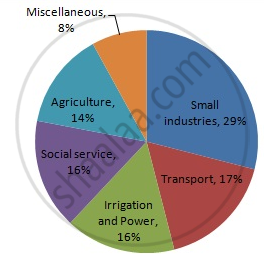Share

# Draw a Pie-diagram for the Following Data of the Investment Pattern in a Five Year Plan: - Mathematics

Course
ConceptCircle Graph Or Pie Chart - Drawing Pie Charts

#### Question

Draw a pie-diagram for the following data of the investment pattern in a five year plan:

 Agriculture Irrigation and Power Small Industries Transport Social service Miscellaneous 14% 16% 29% 17% 16% 8%

#### Solution

We know:
Central angle of a component = (component value/sum of component values x 360)
Here the total percentage = 100%
Thus, the central angle for each component can be calculated as follows:

 Item Amount(in %) Sector angle Agriculture 14 14/100 x 360 = 50.4 Irrigation and Power 16 16/100 x 360 = 57.6 Small Industries 29 29/100 x 360 = 104.4 Transport 17 17/100 x 360 = 61.2 Social Service 16 16/100 x 360 = 57.6 Miscellaneous 8 8/100 x 360 = 28.8

Now, the pie chat representing the given data can be constructed by following the steps below:
Step 1 : Draw circle of an appropriate radius.
Step 2 : Draw a vertical radius of the circle drawn in step 1.
Step 3 : Choose the largest central angle. Here the largest central angle is 104.4o. Draw a sector with the central angle 104.4o in such a way that one of its radii coincides with the radius drawn in step 2 and another radius is in its counter clockwise direction.
Step 4 : Construct the other sectors representing the other items in the clockwise sense in descending order of magnitudes of their central angles.
​Step 5 : Shade the sectors with different colours and label them as shown in the figure below.​​Is there an error in this question or solution?

#### APPEARS IN

RD Sharma Solution for Mathematics for Class 8 by R D Sharma (2019-2020 Session) (2017 to Current)
Chapter 25: Data Handling-III (Pictorial Representation of Data as Pie Charts or Circle Graphs)
Ex. 25.1 | Q: 17 | Page no. 14

#### Video TutorialsVIEW ALL 

Solution Draw a Pie-diagram for the Following Data of the Investment Pattern in a Five Year Plan: Concept: Circle Graph Or Pie Chart - Drawing Pie Charts.
S